FreeFEM Documentation on GitHub

stars - forks

# Static problems

## Soap Film

Our starting point here will be the mathematical model to find the shape of soap film which is glued to the ring on the $$xy-$$plane:

$C=\{(x,y);\;x=\cos t,\,y=\sin t,\,0\leq t\leq 2\pi \}$

We assume the shape of the film is described by the graph $$(x,y,u(x,y))$$ of the vertical displacement $$u(x,y)\, (x^2+y^2<1)$$ under a vertical pressure $$p$$ in terms of force per unit area and an initial tension $$\mu$$ in terms of force per unit length.

Consider the “small plane” ABCD, A:$$(x,y,u(x,y))$$, B:$$(x,y,u(x+\delta x,y))$$, C:$$(x,y,u(x+\delta x,y+\delta y))$$ and D:$$(x,y,u(x,y+\delta y))$$.

Denote by $$\vec{n}(x,y)=(n_x(x,y),n_y(x,y),n_z(x,y))$$ the normal vector of the surface $$z=u(x,y)$$. We see that the vertical force due to the tension $$\mu$$ acting along the edge AD is $$-\mu n_x(x,y)\delta y$$ and the the vertical force acting along the edge AD is:

$\mu n_x(x+\delta x,y)\delta y\simeq \mu\left(n_x(x,y)+\frac{\p n_x}{\p x}\delta x\right)(x,y)\delta y$

Similarly, for the edges AB and DC we have:

$-\mu n_y(x,y)\delta x,\quad\mu\left(n_y(x,y)+\p n_y/\p y\right)(x,y)\delta x$

The force in the vertical direction on the surface ABCD due to the tension $$\mu$$ is given by:

$\mu\left(\p n_x/\p x\right)\delta x\delta y+T\left(\p n_y/\p y\right)\delta y\delta x$

Assuming small displacements, we have:

$\begin{split}\begin{array}{rcccl} \nu_x&=&(\p u/\p x)/\sqrt{1+(\p u/\p x)^2+(\p u/\p y)^2}&\simeq& \p u/\p x,\\ \nu_y&=&(\p u/\p y)/\sqrt{1+(\p u/\p x)^2+(\p u/\p y)^2}&\simeq& \p u/\p y \end{array}\end{split}$

Letting $$\delta x\to dx,\, \delta y\to dy$$, we have the equilibrium of the vertical displacement of soap film on ABCD by $$p$$:

$\mu dx dy\p^2 u/\p x^2 +\mu dx dy\p^2 u/\p y^2 + p dx dy = 0$

Using the Laplace operator $$\Delta = \p^2 /\p x^2 + \p^2 /\p y^2$$, we can find the virtual displacement write the following:

$-\Delta u = f\quad \mbox{in }\Omega$

where $$f=p/\mu$$, $$\Omega =\{(x,y);\;x^{2}+y^{2}<1\}$$.

Poisson’s equation appears also in electrostatics taking the form of $$f=\rho / \epsilon$$ where $$\rho$$ is the charge density, $$\epsilon$$ the dielectric constant and $$u$$ is named as electrostatic potential.

The soap film is glued to the ring $$\p \Omega =C$$, then we have the boundary condition:

$u=0\quad \mbox{on }\p \Omega$

If the force is gravity, for simplify, we assume that $$f=-1$$.

 1// Parameters
2int nn = 50;
3func f = -1;
4func ue = (x^2+y^2-1)/4; //ue: exact solution
5
6// Mesh
7border a(t=0, 2*pi){x=cos(t); y=sin(t); label=1;}
8mesh disk = buildmesh(a(nn));
9plot(disk);
10
11// Fespace
12fespace femp1(disk, P1);
13femp1 u, v;
14
15// Problem
16problem laplace (u, v)
17    = int2d(disk)( //bilinear form
18        dx(u)*dx(v)
19        + dy(u)*dy(v)
20    )
21    - int2d(disk)( //linear form
22        f*v
23    )
24    + on(1, u=0) //boundary condition
25    ;
26
27// Solve
28laplace;
29
30// Plot
31plot (u, value=true, wait=true);
32
33// Error
34femp1 err = u - ue;
35plot(err, value=true, wait=true);
36
37cout << "error L2 = " << sqrt( int2d(disk)(err^2) )<< endl;
38cout << "error H10 = " << sqrt( int2d(disk)((dx(u)-x/2)^2) + int2d(disk)((dy(u)-y/2)^2) )<< endl;
39
40/// Re-run with a mesh adaptation ///
41
44plot(disk, wait=true);
45
46// Solve
47laplace;
48plot (u, value=true, wait=true);
49
50// Error
51err = u - ue; //become FE-function on adapted mesh
52plot(err, value=true, wait=true);
53
54cout << "error L2 = " << sqrt( int2d(disk)(err^2) )<< endl;
55cout << "error H10 = " << sqrt( int2d(disk)((dx(u)-x/2)^2) + int2d(disk)((dy(u)-y/2)^2) )<< endl;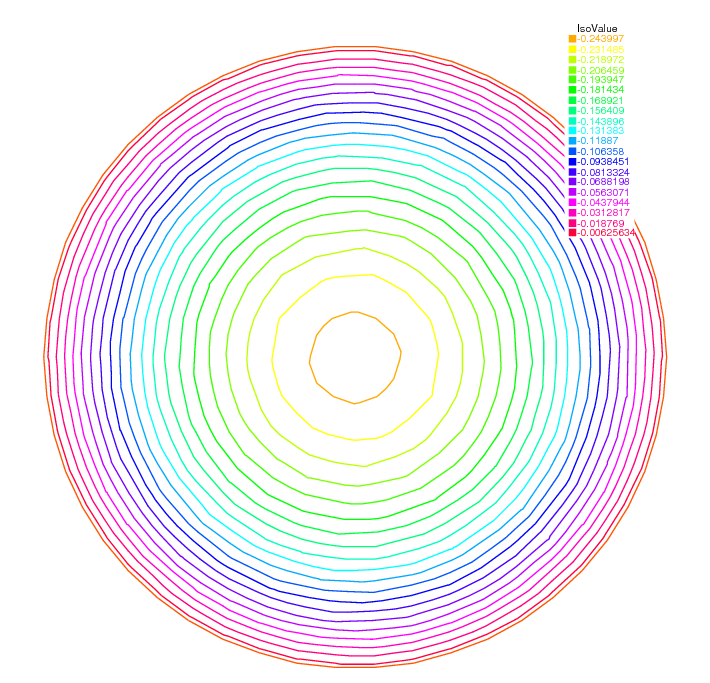Fig. 136 Isovalue of $$u$$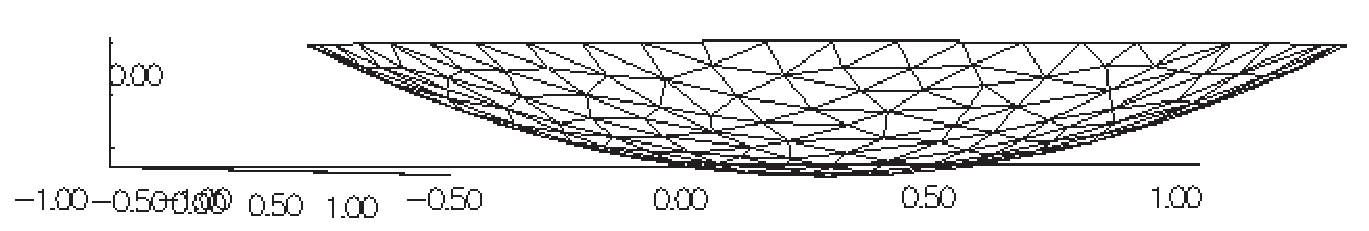Fig. 137 A side view of $$u$$

In the 37th line, the $$L^2$$-error estimation between the exact solution $$u_e$$,

$\|u_h - u_e\|_{0,\Omega}=\left(\int_{\Omega}|u_h-u_e|^2\, \d x\d y\right)^{1/2}$

and in the following line, the $$H^1$$-error seminorm estimation:

$|u_h - u_e|_{1,\Omega}=\left(\int_{\Omega}|\nabla u_h-\nabla u_e|^2\, \d x\d y\right)^{1/2}$

are done on the initial mesh. The results are $$\|u_h - u_e\|_{0,\Omega}=0.000384045,\, |u_h - u_e|_{1,\Omega}=0.0375506$$.

After the adaptation, we have $$\|u_h - u_e\|_{0,\Omega}=0.000109043,\, |u_h - u_e|_{1,\Omega}=0.0188411$$. So the numerical solution is improved by adaptation of mesh.

## Electrostatics

We assume that there is no current and a time independent charge distribution. Then the electric field $$\mathbf{E}$$ satisfies:

(55)$\begin{split}\begin{array}{rcl} \mathrm{div}\mathbf{E} &=& \rho/\epsilon\\ \mathrm{curl}\mathbf{E} &=& 0 \end{array}\end{split}$

where $$\rho$$ is the charge density and $$\epsilon$$ is called the permittivity of free space.

From the equation (55) We can introduce the electrostatic potential such that $$\mathbf{E}=-\nabla \phi$$. Then we have Poisson’s equation $$-\Delta \phi=f$$, $$f=-\rho/\epsilon$$.

We now obtain the equipotential line which is the level curve of $$\phi$$, when there are no charges except conductors $$\{C_i\}_{1,\cdots,K}$$. Let us assume $$K$$ conductors $$C_1,\cdots,C_K$$ within an enclosure $$C_0$$.

Each one is held at an electrostatic potential $$\varphi_i$$. We assume that the enclosure $$C0$$ is held at potential 0. In order to know $$\varphi(x)$$ at any point $$x$$ of the domain $$\Omega$$, we must solve:

$-\Delta \varphi =0\quad \textrm{ in }\Omega$

where $$\Omega$$ is the interior of $$C_0$$ minus the conductors $$C_i$$, and $$\Gamma$$ is the boundary of $$\Omega$$, that is $$\sum_{i=0}^N C_i$$.

Here $$g$$ is any function of $$x$$ equal to $$\varphi_i$$ on $$C_i$$ and to 0 on $$C_0$$. The boundary equation is a reduced form for:

$\varphi =\varphi_{i}\;\text{on }C_{i},\;i=1...N,\varphi =0\;\text{on }C_{0}.$

First we give the geometrical informations; $$C_0=\{(x,y);\; x^2+y^2=5^2\}$$, $$C_1=\{(x,y):\;\frac{1}{0.3^2}(x-2)^2+\frac{1}{3^2}y^2=1\}$$, $$C_2=\{(x,y):\; \frac{1}{0.3^2}(x+2)^2+\frac{1}{3^2}y^2=1\}$$.

Let $$\Omega$$ be the disk enclosed by $$C_0$$ with the elliptical holes enclosed by $$C_1$$ and $$C_2$$. Note that $$C_0$$ is described counterclockwise, whereas the elliptical holes are described clockwise, because the boundary must be oriented so that the computational domain is to its left.

 1// Mesh
2border C0(t=0, 2*pi){x=5*cos(t); y=5*sin(t);}
3border C1(t=0, 2*pi){x=2+0.3*cos(t); y=3*sin(t);}
4border C2(t=0, 2*pi){x=-2+0.3*cos(t); y=3*sin(t);}
5
6mesh Th = buildmesh(C0(60) + C1(-50) + C2(-50));
7plot(Th);
8
9// Fespace
10fespace Vh(Th, P1);
11Vh uh, vh;
12
13// Problem
14problem Electro (uh, vh)
15    = int2d(Th)( //bilinear
16        dx(uh)*dx(vh)
17        + dy(uh)*dy(vh)
18    )
19    + on(C0, uh=0) //boundary condition on C_0
20    + on(C1, uh=1) //+1 volt on C_1
21    + on(C2, uh=-1) //-1 volt on C_2
22    ;
23
24// Solve
25Electro;
26plot(uh);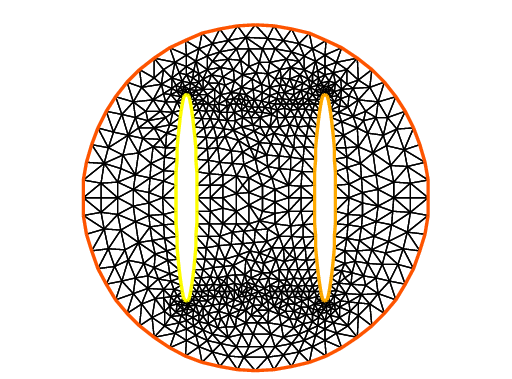Fig. 138 Disk with two elliptical holes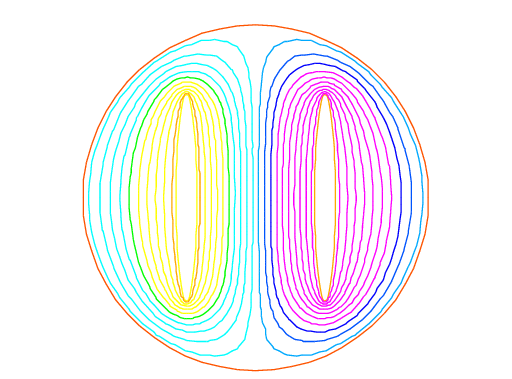Fig. 139 Equipotential lines where $$C_1$$ is located in right hand side

## Aerodynamics

Let us consider a wing profile $$S$$ in a uniform flow. Infinity will be represented by a large circle $$\Gamma_{\infty}$$. As previously, we must solve:

(56)$\Delta \varphi=0\quad\textrm{in }\Omega, \quad \varphi|_S=c,\quad \varphi|_{\Gamma_{\infty}}=u_{\infty 1x}-u_{\infty2x}$

where $$\Omega$$ is the area occupied by the fluid, $$u_{\infty}$$ is the air speed at infinity, $$c$$ is a constant to be determined so that $$\p_n\varphi$$ is continuous at the trailing edge $$P$$ of $$S$$ (so-called Kutta-Joukowski condition). Lift is proportional to $$c$$.

To find $$c$$ we use a superposition method. As all equations in (56) are linear, the solution $$\varphi_c$$ is a linear function of $$c$$

$\varphi_c = \varphi_0 + c\varphi_1$

where $$\varphi_0$$ is a solution of (56) with $$c = 0$$ and $$\varphi_1$$ is a solution with $$c = 1$$ and zero speed at infinity.

With these two fields computed, we shall determine $$c$$ by requiring the continuity of $$\p \varphi /\p n$$ at the trailing edge. An equation for the upper surface of a NACA0012 (this is a classical wing profile in aerodynamics; the rear of the wing is called the trailing edge) is:

$y = 0.17735\sqrt{x} - 0.075597x - 0.212836x^2 + 0.17363x^3 - 0.06254x^4$

Taking an incidence angle $$\alpha$$ such that $$\tan \alpha = 0.1$$, we must solve:

$-\Delta\varphi = 0\qquad \textrm{in }\Omega, \quad \varphi|_{\Gamma_1} = y - 0.1x,\quad \varphi |_{\Gamma_2} = c$

where $$\Gamma_2$$ is the wing profile and $$\Gamma_1$$ is an approximation of infinity. One finds $$c$$ by solving:

$\begin{split}\begin{array}{ccccccc} -\Delta\varphi_0 &= 0 &\textrm{in }\Omega&,\qquad \varphi_0|_{\Gamma_1} &= y - 0.1x&, \quad \varphi_0|_{\Gamma_2} &= 0,\\ -\Delta\varphi_1 &= 0 &\textrm{in }\Omega&, \qquad \varphi_1|_{\Gamma_1} &= 0&, \quad \varphi_1|_{\Gamma_2} &= 1 \end{array}\end{split}$

The solution $$\varphi = \varphi_0+c\varphi_1$$ allows us to find $$c$$ by writing that $$\p_n\varphi$$ has no jump at the trailing edge $$P = (1, 0)$$.

We have $$\p n\varphi -(\varphi (P^+)-\varphi (P))/\delta$$ where $$P^+$$ is the point just above $$P$$ in the direction normal to the profile at a distance $$\delta$$. Thus the jump of $$\p_n\varphi$$ is $$(\varphi_0|_{P^+} +c(\varphi_1|_{P^+} -1))+(\varphi_0|_{P^-} +c(\varphi_1|_{P^-} -1))$$ divided by $$\delta$$ because the normal changes sign between the lower and upper surfaces. Thus

$c = -\frac{\varphi_0|_{P^+} + \varphi_0|_{P^-}} {(\varphi_1|_{P^+} + \varphi_1|_{P^-} - 2)} ,$

which can be programmed as:

$c = -\frac{\varphi_0(0.99, 0.01) + \varphi_0(0.99,-0.01)} {(\varphi_1(0.99, 0.01) + \varphi_1(0.99,-0.01) - 2)} .$
 1// Mesh
2border a(t=0, 2*pi){x=5*cos(t); y=5*sin(t);}
3border upper(t=0, 1) {
4    x=t;
5    y=0.17735*sqrt(t)-0.075597*t - 0.212836*(t^2) + 0.17363*(t^3) - 0.06254*(t^4);
6}
7border lower(t=1, 0) {
8    x=t;
9    y=-(0.17735*sqrt(t) - 0.075597*t - 0.212836*(t^2) + 0.17363*(t^3) - 0.06254*(t^4));
10}
11border c(t=0, 2*pi){x=0.8*cos(t)+0.5; y=0.8*sin(t);}
12
13mesh Zoom = buildmesh(c(30) + upper(35) + lower(35));
14mesh Th = buildmesh(a(30) + upper(35) + lower(35));
15
16// Fespace
17fespace Vh(Th, P2);
18Vh psi0, psi1, vh;
19
20fespace ZVh(Zoom, P2);
21
22// Problem
23solve Joukowski0(psi0, vh)
24    = int2d(Th)(
25        dx(psi0)*dx(vh)
26        + dy(psi0)*dy(vh)
27    )
28    + on(a, psi0=y-0.1*x)
29    + on(upper, lower, psi0=0)
30    ;
31
32plot(psi0);
33
34solve Joukowski1(psi1,vh)
35    = int2d(Th)(
36        dx(psi1)*dx(vh)
37        + dy(psi1)*dy(vh)
38    )
39    + on(a, psi1=0)
40    + on(upper, lower, psi1=1);
41
42plot(psi1);
43
44//continuity of pressure at trailing edge
45real beta = psi0(0.99,0.01) + psi0(0.99,-0.01);
46beta = -beta / (psi1(0.99,0.01) + psi1(0.99,-0.01)-2);
47
48Vh psi = beta*psi1 + psi0;
49plot(psi);
50
51ZVh Zpsi = psi;
52plot(Zpsi, bw=true);
53
54ZVh cp = -dx(psi)^2 - dy(psi)^2;
55plot(cp);
56
57ZVh Zcp = cp;
58plot(Zcp, nbiso=40);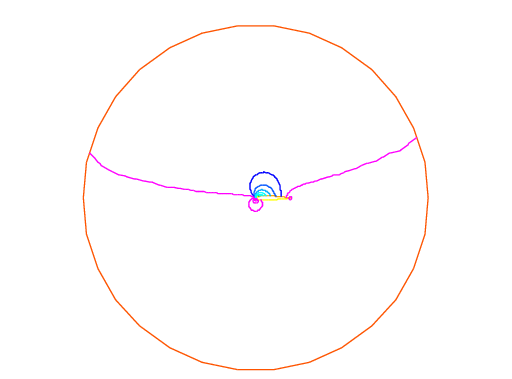Fig. 140 Isovalue of $$cp = -(\p_x\psi)^2 - (\p_y\psi)^2$$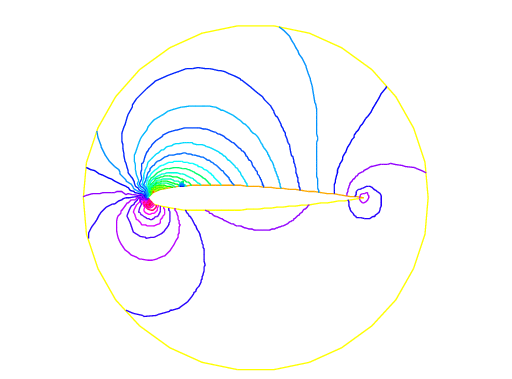Fig. 141 Zooming of $$cp$$

## Error estimation

There are famous estimation between the numerical result $$u_h$$ and the exact solution $$u$$ of the Poisson’s problem:

If triangulations $$\{\mathcal{T}_h\}_{h\downarrow 0}$$ is regular (see Regular Triangulation), then we have the estimates:

(57)$\begin{split}\begin{array}{rcl} |\nabla u - \nabla u_h|_{0,\Omega} &\le& C_1h \\ \|u - u_h\|_{0,\Omega} &\le& C_2h^2 \end{array}\end{split}$

with constants $$C_1,\, C_2$$ independent of $$h$$, if $$u$$ is in $$H^2(\Omega)$$. It is known that $$u\in H^2(\Omega)$$ if $$\Omega$$ is convex.

In this section we check (57). We will pick up numericall error if we use the numerical derivative, so we will use the following for (57).

$\begin{split}\begin{array}{rcl} \int_{\Omega}|\nabla u - \nabla u_h|^2\, \d x\d y &=&\int_{\Omega}\nabla u\cdot \nabla(u - 2u_h)\, \d x\d y+ \int_{\Omega}\nabla u_h\cdot \nabla u_h\, \d x\d y\\ &=&\int_{\Omega}f(u-2u_h)\, \d x\d y+\int_{\Omega}fu_h\, \d x\d y \end{array}\end{split}$

The constants $$C_1,\, C_2$$ are depend on $$\mathcal{T}_h$$ and $$f$$, so we will find them by FreeFEM.

In general, we cannot get the solution $$u$$ as a elementary functions even if spetical functions are added. Instead of the exact solution, here we use the approximate solution $$u_0$$ in $$V_h(\mathcal{T}_h,P_2),\, h\sim 0$$.

 1// Parameters
2func f = x*y;
3
4//Mesh
5mesh Th0 = square(100, 100);
6
7// Fespace
8fespace V0h(Th0, P2);
9V0h u0, v0;
10
11// Problem
12solve Poisson0 (u0, v0)
13    = int2d(Th0)(
14        dx(u0)*dx(v0)
15        + dy(u0)*dy(v0)
16    )
17    - int2d(Th0)(
18        f*v0
19    )
20    + on(1, 2, 3, 4, u0=0)
21    ;
22plot(u0);
23
24// Error loop
25real[int] errL2(10), errH1(10);
26for (int i = 1; i <= 10; i++){
27    // Mesh
28    mesh Th = square(5+i*3,5+i*3);
29
30    // Fespace
31    fespace Vh(Th, P1);
32    Vh u, v;
33    fespace Ph(Th, P0);
34    Ph h = hTriangle; //get the size of all triangles
35
36    // Problem
37    solve Poisson (u, v)
38        = int2d(Th)(
39            dx(u)*dx(v)
40            + dy(u)*dy(v)
41        )
42        - int2d(Th)(
43            f*v
44        )
45        + on(1, 2, 3, 4, u=0)
46        ;
47
48    // Error
49    V0h uu = u; //interpolate solution on first mesh
50    errL2[i-1] = sqrt( int2d(Th0)((uu - u0)^2) )/h[].max^2;
51    errH1[i-1] = sqrt( int2d(Th0)(f*(u0 - 2*uu + uu)) )/h[].max;
52}
53
54// Display
55cout << "C1 = " << errL2.max << "("<<errL2.min<<")" << endl;
56cout << "C2 = " << errH1.max << "("<<errH1.min<<")" << endl;


We can guess that $$C_1=0.0179253(0.0173266)$$ and $$C_2=0.0729566(0.0707543)$$, where the numbers inside the parentheses are minimum in calculation.

## Periodic Boundary Conditions

We now solve the Poisson equation:

$-\Delta u = sin(x+\pi/4.)*cos(y+\pi/4.)$

on the square $$]0,2\pi[^2$$ under bi-periodic boundary condition $$u(0,y)=u(2\pi,y)$$ for all $$y$$ and $$u(x,0)=u(x,2\pi)$$ for all $$x$$.

These boundary conditions are achieved from the definition of the periodic finite element space.

 1// Parameters
2func f = sin(x+pi/4.)*cos(y+pi/4.); //right hand side
3
4// Mesh
5mesh Th = square(10, 10, [2*x*pi, 2*y*pi]);
6
7// Fespace
8//defined the fespace with periodic condition
9//label: 2 and 4 are left and right side with y the curve abscissa
10//       1 and 2 are bottom and upper side with x the curve abscissa
11fespace Vh(Th, P2, periodic=[[2, y], [4, y], [1, x], [3, x]]);
12Vh uh, vh;
13
14// Problem
15problem laplace (uh, vh)
16    = int2d(Th)(
17        dx(uh)*dx(vh)
18        + dy(uh)*dy(vh)
19    )
20    + int2d(Th)(
21        - f*vh
22    )
23    ;
24
25// Solve
26laplace;
27
28// Plot
29plot(uh, value=true);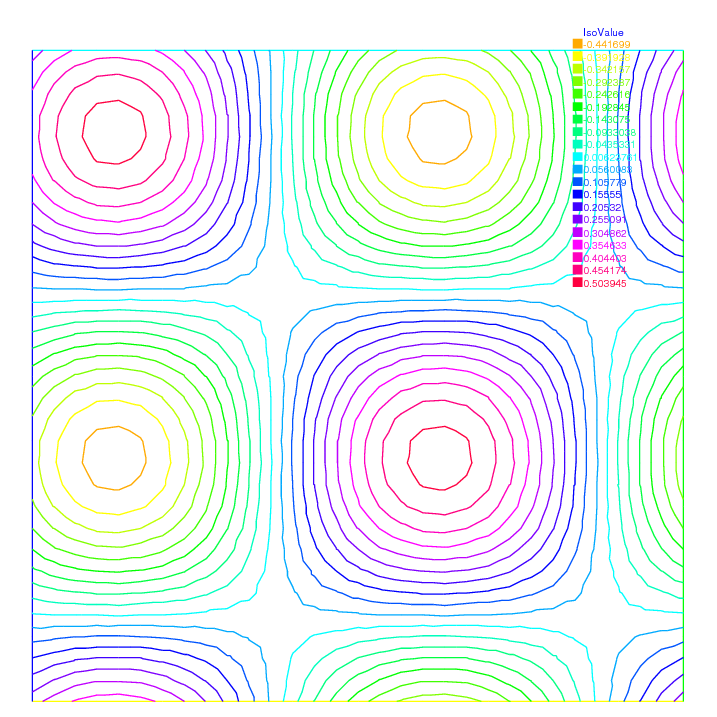Fig. 142 The isovalue of solution $$u$$ with periodic boundary condition

The periodic condition does not necessarily require parallel boundaries. The following example give such example.

Tip

Periodic boundary conditions - non-parallel boundaries

 1// Parameters
2int n = 10;
3real r = 0.25;
4real r2 = 1.732;
5func f = (y+x+1)*(y+x-1)*(y-x+1)*(y-x-1);
6
7// Mesh
8border a(t=0, 1){x=-t+1; y=t; label=1;};
9border b(t=0, 1){x=-t; y=1-t; label=2;};
10border c(t=0, 1){x=t-1; y=-t; label=3;};
11border d(t=0, 1){x=t; y=-1+t; label=4;};
12border e(t=0, 2*pi){x=r*cos(t); y=-r*sin(t); label=0;};
13mesh Th = buildmesh(a(n) + b(n) + c(n) + d(n) + e(n));
14plot(Th, wait=true);
15
16// Fespace
17//warning for periodic condition:
18//side a and c
19//on side a (label 1) $x \in [0,1]$ or $x-y\in [-1,1]$
20//on side c (label 3) $x \in [-1,0]$ or $x-y\in[-1,1]$
21//so the common abscissa can be respectively $x$ and $x+1$
22//or you can can try curviline abscissa $x-y$ and $x-y$
23//1 first way
24//fespace Vh(Th, P2, periodic=[[2, 1+x], [4, x], [1, x], [3, 1+x]]);
25//2 second way
26fespace Vh(Th, P2, periodic=[[2, x+y], [4, x+y], [1, x-y], [3, x-y]]);
27Vh uh, vh;
28
29// Problem
30real intf = int2d(Th)(f);
31real mTh = int2d(Th)(1);
32real k =  intf / mTh;
33problem laplace (uh, vh)
34    = int2d(Th)(
35        dx(uh)*dx(vh)
36        + dy(uh)*dy(vh)
37    )
38    + int2d(Th)(
39        (k-f)*vh
40    )
41    ;
42
43// Solve
44laplace;
45
46// Plot
47plot(uh, wait=true);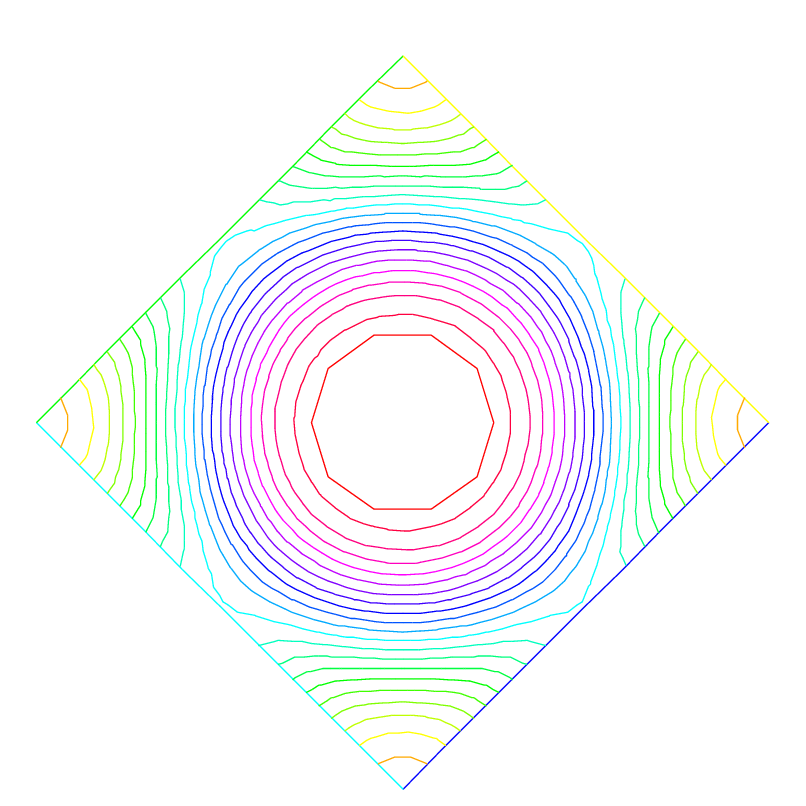Fig. 143 The isovalue of solution $$u$$ for $$\Delta u = ((y+x)^{2}+1)((y-x)^{2}+1) - k$$, in $$\Omega$$ and $$\p_{n} u =0$$ on hole, and with two periodic boundary condition on external border

An other example with no equal border, just to see if the code works.

Tip

Periodic boundary conditions - non-equal border

 1// Macro
2//irregular boundary condition to build border AB
3macro LINEBORDER(A, B, lab)
4    border A#B(t=0,1){ real t1=1.-t;
5    x=A#x*t1+B#x*t;
6    y=A#y*t1+B#y*t;
7    label=lab; } //EOM
8// compute \||AB|\| A=(ax,ay) et B =(bx,by)
9macro dist(ax, ay, bx, by)
10    sqrt(square((ax)-(bx)) + square((ay)-(by))) //EOM
12
13// Parameters
14int n = 10;
15real Ax = 0.9, Ay = 1;
16real Bx = 2, By = 1;
17real Cx = 2.5, Cy = 2.5;
18real Dx = 1, Dy = 2;
19real gx = (Ax+Bx+Cx+Dx)/4.;
20real gy = (Ay+By+Cy+Dy)/4.;
21
22// Mesh
23LINEBORDER(A,B,1)
24LINEBORDER(B,C,2)
25LINEBORDER(C,D,3)
26LINEBORDER(D,A,4)
27mesh Th=buildmesh(AB(n)+BC(n)+CD(n)+DA(n),fixedborder=1);
28
29// Fespace
30real l1 = dist(Ax,Ay,Bx,By);
31real l2 = dist(Bx,By,Cx,Cy);
32real l3 = dist(Cx,Cy,Dx,Dy);
33real l4 = dist(Dx,Dy,Ax,Ay);
34func s1 = dist(Ax,Ay,x,y)/l1; //absisse on AB = ||AX||/||AB||
35func s2 = dist(Bx,By,x,y)/l2; //absisse on BC = ||BX||/||BC||
36func s3 = dist(Cx,Cy,x,y)/l3; //absisse on CD = ||CX||/||CD||
37func s4 = dist(Dx,Dy,x,y)/l4; //absisse on DA = ||DX||/||DA||
38verbosity = 6; //to see the abscisse value of the periodic condition
39fespace Vh(Th, P1, periodic=[[1, s1], [3, s3], [2, s2], [4, s4]]);
40verbosity = 1; //reset verbosity
41Vh u, v;
42
43real cc = 0;
44cc = int2d(Th)((x-gx)*(y-gy)-cc)/Th.area;
45cout << "compatibility = " << int2d(Th)((x-gx)*(y-gy)-cc) <<endl;
46
47// Problem
48solve Poisson (u, v)
49    = int2d(Th)(
51        + 1e-10*u*v
52    )
53    -int2d(Th)(
54        10*v*((x-gx)*(y-gy)-cc)
55    )
56    ;
57
58// Plot
59plot(u, value=true);


Tip

Periodic boundry conditions - Poisson cube-balloon

 1load "msh3" load "tetgen" load "medit"
2
3// Parameters
4real hs = 0.1; //mesh size on sphere
5int[int] N = [20, 20, 20];
6real [int,int] B = [[-1, 1], [-1, 1], [-1, 1]];
7int [int,int] L = [[1, 2], [3, 4], [5, 6]];
8
9real x0 = 0.3, y0 = 0.4, z0 = 06;
10func f = sin(x*2*pi+x0)*sin(y*2*pi+y0)*sin(z*2*pi+z0);
11
12// Mesh
13bool buildTh = 0;
14mesh3 Th;
15try { //a way to build one time the mesh or read it if the file exist
17}
18catch (...){
19    buildTh = 1;
20}
21
22if (buildTh){
23    include "MeshSurface.idp"
24
25    // Surface Mesh
26    mesh3 ThH = SurfaceHex(N, B, L, 1);
27    mesh3 ThS = Sphere(0.5, hs, 7, 1);
28
29    mesh3 ThHS = ThH + ThS;
30
31    real voltet = (hs^3)/6.;
32    real[int] domain = [0, 0, 0, 1, voltet, 0, 0, 0.7, 2, voltet];
33    Th = tetg(ThHS, switch="pqaAAYYQ", nbofregions=2, regionlist=domain);
34
35    savemesh(Th, "Th-hex-sph.mesh");
36}
37
38// Fespace
39fespace Ph(Th, P0);
40Ph reg = region;
41cout << " centre = " << reg(0,0,0) << endl;
42cout << " exterieur = " << reg(0,0,0.7) << endl;
43
44verbosity = 50;
45fespace Vh(Th, P1, periodic=[[3, x, z], [4, x, z], [1, y, z], [2, y, z], [5, x, y], [6, x, y]]);
46verbosity = 1;
47Vh uh,vh;
48
49// Macro
51
52// Problem
53problem Poisson (uh, vh)
54    = int3d(Th, 1)(
56    )
57    + int3d(Th, 2)(
59    )
60    + int3d(Th)(
61        vh*f
62    )
63    ;
64
65// Solve
66Poisson;
67
68// Plot
69plot(uh, wait=true, nbiso=6);
70medit("uh", Th, uh);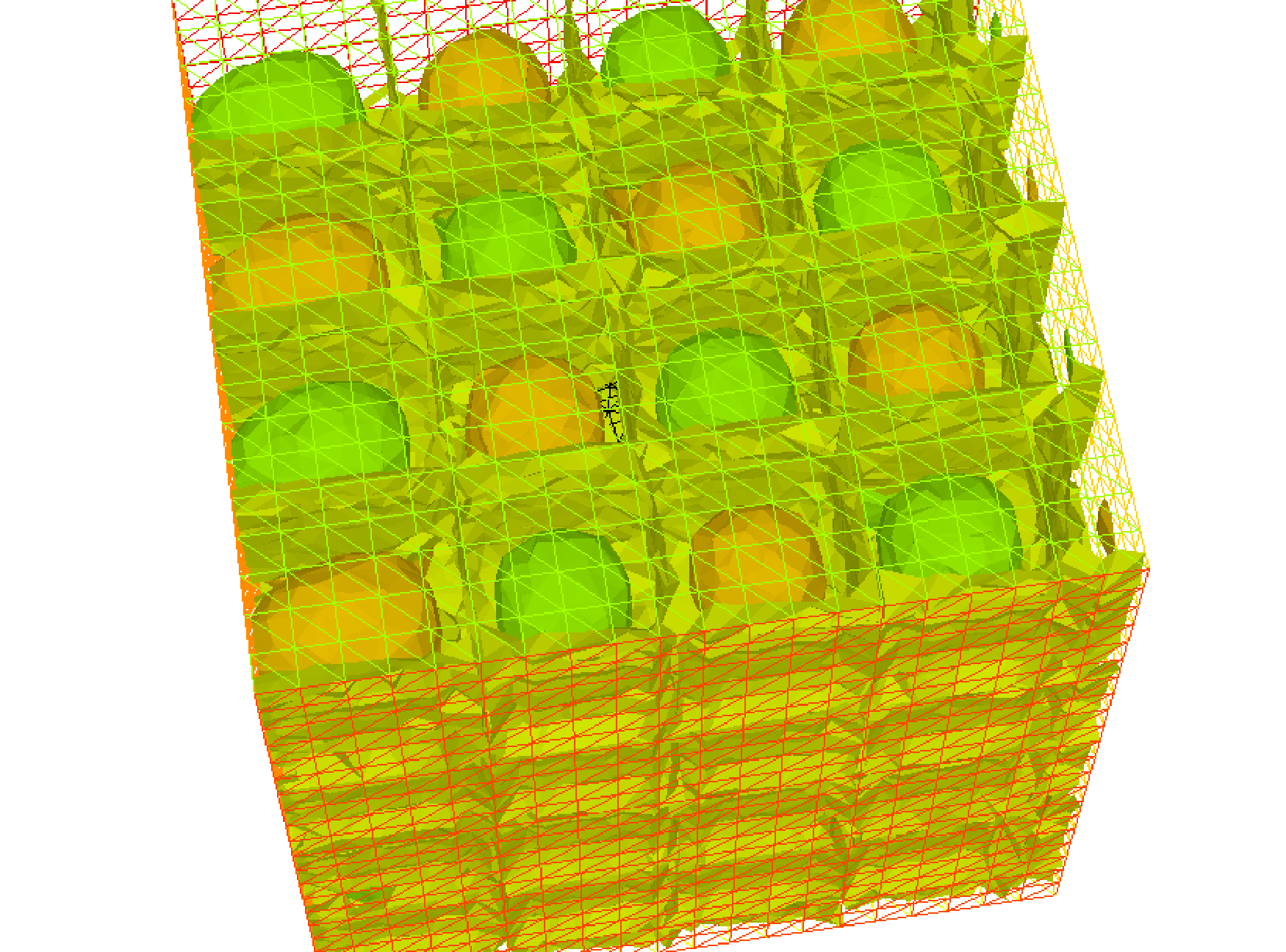Fig. 144 View of the surface isovalue of periodic solution $$uh$$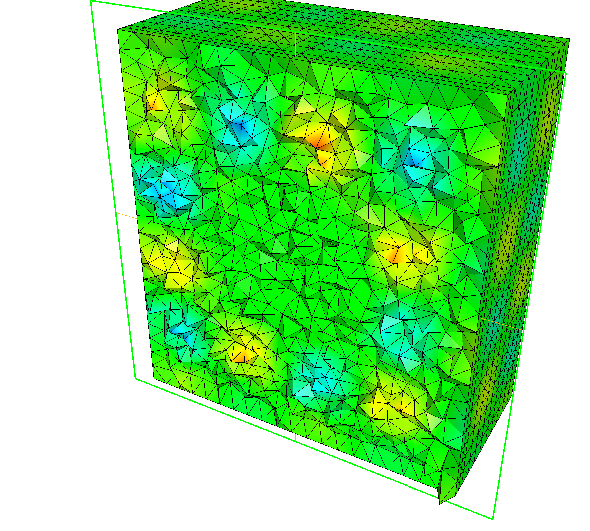Fig. 145 View a the cut of the solution $$uh$$ with ffmedit

## Poisson Problems with mixed boundary condition

Here we consider the Poisson equation with mixed boundary conditions:

For given functions $$f$$ and $$g$$, find $$u$$ such that:

$\begin{split}\begin{array}{rcll} -\Delta u &=& f & \textrm{ in }\Omega\\ u &=& g &\textrm{ on }\Gamma_D\\ \p u/\p n &=& 0 &\textrm{ on }\Gamma_N \end{array}\end{split}$

where $$\Gamma_D$$ is a part of the boundary $$\Gamma$$ and $$\Gamma_N=\Gamma\setminus \overline{\Gamma_D}$$.

The solution $$u$$ has the singularity at the points $$\{\gamma_1,\gamma_2\}=\overline{\Gamma_D}\cap\overline{\Gamma_N}$$.

When $$\Omega=\{(x,y);\; -1<x<1,\, 0<y<1\}$$, $$\Gamma_N=\{(x,y);\; -1\le x<0,\, y=0\}$$, $$\Gamma_D=\p \Omega\setminus \Gamma_N$$, the singularity will appear at $$\gamma_1=(0,0),\, \gamma_2(-1,0)$$, and $$u$$ has the expression:

$u=K_iu_S + u_R,\, u_R\in H^2(\textrm{near }\gamma_i),\, i=1,2$

with a constants $$K_i$$.

Here $$u_S = r_j^{1/2}\sin(\theta_j/2)$$ by the local polar coordinate $$(r_j,\theta_j$$ at $$\gamma_j$$ such that $$(r_1,\theta_1)=(r,\theta)$$.

Instead of polar coordinate system $$(r,\theta)$$, we use that $$r$$ = sqrt ($$x^2+y^2$$) and $$\theta$$ = atan2 ($$y,x$$) in FreeFEM.

Assume that $$f=-2\times 30(x^2+y^2)$$ and $$g=u_e=10(x^2+y^2)^{1/4}\sin\left([\tan^{-1}(y/x)]/2\right)+30(x^2y^2)$$, where $$u_e$$S is the exact solution.

 1// Parameters
2func f = -2*30*(x^2+y^2); //given function
3//the singular term of the solution is K*us (K: constant)
4func us = sin(atan2(y,x)/2)*sqrt( sqrt(x^2+y^2) );
5real K = 10.;
6func ue = K*us + 30*(x^2*y^2);
7
8// Mesh
9border N(t=0, 1){x=-1+t; y=0; label=1;};
10border D1(t=0, 1){x=t; y=0; label=2;};
11border D2(t=0, 1){x=1; y=t; label=2;};
12border D3(t=0, 2){x=1-t; y=1; label=2;};
13border D4(t=0, 1){x=-1; y=1-t; label=2;};
14
15mesh T0h = buildmesh(N(10) + D1(10) + D2(10) + D3(20) + D4(10));
16plot(T0h, wait=true);
17
18// Fespace
19fespace V0h(T0h, P1);
20V0h u0, v0;
21
22//Problem
23solve Poisson0 (u0, v0)
24    = int2d(T0h)(
25        dx(u0)*dx(v0)
26        + dy(u0)*dy(v0)
27    )
28    - int2d(T0h)(
29        f*v0
30    )
31    + on(2, u0=ue)
32    ;
33
34// Mesh adaptation by the singular term
36for (int i = 0; i < 5; i++)
38
39// Fespace
40fespace Vh(Th, P1);
41Vh u, v;
42
43// Problem
44solve Poisson (u, v)
45    = int2d(Th)(
46        dx(u)*dx(v)
47        + dy(u)*dy(v)
48    )
49    - int2d(Th)(
50        f*v
51    )
52    + on(2, u=ue)
53    ;
54
55// Plot
56plot(Th);
57plot(u, wait=true);
58
59// Error in H1 norm
60Vh uue = ue;
61real H1e = sqrt( int2d(Th)(dx(uue)^2 + dy(uue)^2 + uue^2) );
62Vh err0 = u0 - ue;
63Vh err = u - ue;
64Vh H1err0 = int2d(Th)(dx(err0)^2 + dy(err0)^2 + err0^2);
65Vh H1err = int2d(Th)(dx(err)^2 + dy(err)^2 + err^2);
66cout << "Relative error in first mesh = "<< int2d(Th)(H1err0)/H1e << endl;
67cout << "Relative error in adaptive mesh = "<< int2d(Th)(H1err)/H1e << endl;


From line 35 to 37, mesh adaptations are done using the base of singular term.

In line 61, H1e = $$|u_e|_{1,\Omega}$$ is calculated.

In lines 64 and 65, the relative errors are calculated, that is:

$\begin{split}\begin{array}{rcl} \|u^0_h-u_e\|_{1,\Omega}/H1e&=&0.120421\\ \|u^a_h-u_e\|_{1,\Omega}/H1e&=&0.0150581 \end{array}\end{split}$

where $$u^0_h$$ is the numerical solution in T0h and $$u^a_h$$ is u in this program.

## Poisson with mixed finite element

Here we consider the Poisson equation with mixed boundary value problems:

For given functions $$f$$ , $$g_d$$, $$g_n$$, find $$p$$ such that

$\begin{split}\begin{array}{rcll} -\Delta p &=& 1 & \textrm{ in }\Omega\\ p &=& g_d & \textrm{ on }\Gamma_D\\ \p p/\p n &=& g_n & \textrm{ on }\Gamma_N \end{array}\end{split}$

where $$\Gamma_D$$ is a part of the boundary $$\Gamma$$ and $$\Gamma_N=\Gamma\setminus \overline{\Gamma_D}$$.

The mixed formulation is: find $$p$$ and $$\mathbf{u}$$ such that:

$\begin{split}\begin{array}{rcll} \nabla p + \mathbf{u} &=& \mathbf{0} & \textrm{ in }\Omega\\ \nabla. \mathbf{u} &=& f & \textrm{ in }\Omega\\ p &=& g_d & \textrm{ on }\Gamma_D\\ \p u. n &=& \mathbf{g}_n.n & \textrm{ on }\Gamma_N \end{array}\end{split}$

where $$\mathbf{g}_n$$ is a vector such that $$\mathbf{g}_n.n = g_n$$.

The variational formulation is:

$\begin{split}\begin{array}{rcl} \forall \mathbf{v} \in \mathbb{V}_0: & \int_\Omega p \nabla.v + \mathbf{v} \mathbf{v} &= \int_{\Gamma_d} g_d \mathbf{v}.n\\ \forall {q} \in \mathbb{P}: & \int_\Omega q \nabla.u &= \int_\Omega q f\nonumber\\ & \p u. n &= \mathbf{g}_n.n \quad \textrm{on }\Gamma_N \end{array}\end{split}$

where the functional space are:

$\mathbb{P}= L^2(\Omega), \qquad\mathbb{V}= H(div)=\{\mathbf{v}\in L^2(\Omega)^2,\nabla.\mathbf{v}\in L^2(\Omega)\}$

and:

$\mathbb{V}_0 = \{\mathbf{v}\in \mathbb{V};\quad\mathbf{v}. n = 0 \quad\mathrm{on }\;\;\Gamma_N\}$

To write the FreeFEM example, we have just to choose the finites elements spaces.

Here $$\mathbb{V}$$ space is discretize with Raviart-Thomas finite element RT0 and $$\mathbb{P}$$ is discretize by constant finite element P0.

Example 9.10 LaplaceRT.edp

 1// Parameters
2func gd = 1.;
3func g1n = 1.;
4func g2n = 1.;
5
6// Mesh
7mesh Th = square(10, 10);
8
9// Fespace
10fespace Vh(Th, RT0);
11Vh [u1, u2];
12Vh [v1, v2];
13
14fespace Ph(Th, P0);
15Ph p, q;
16
17// Problem
18problem laplaceMixte ([u1, u2, p], [v1, v2, q], solver=GMRES, eps=1.0e-10, tgv=1e30, dimKrylov=150)
19    = int2d(Th)(
20        p*q*1e-15 //this term is here to be sure
21        // that all sub matrix are inversible (LU requirement)
22        + u1*v1
23        + u2*v2
24        + p*(dx(v1)+dy(v2))
25        + (dx(u1)+dy(u2))*q
26    )
27    + int2d(Th) (
28        q
29    )
30    - int1d(Th, 1, 2, 3)(
31        gd*(v1*N.x +v2*N.y)
32    )
33    + on(4, u1=g1n, u2=g2n)
34    ;
35
36// Solve
37laplaceMixte;
38
39// Plot
40plot([u1, u2], coef=0.1, wait=true, value=true);
41plot(p, fill=1, wait=true, value=true);


## Metric Adaptation and residual error indicator

We do metric mesh adaption and compute the classical residual error indicator $$\eta_{T}$$ on the element $$T$$ for the Poisson problem.

First, we solve the same problem as in a previous example.

 1// Parameters
2real[int] viso(21);
3for (int i = 0; i < viso.n; i++)
4viso[i] = 10.^(+(i-16.)/2.);
5real error = 0.01;
6func f = (x-y);
7
8// Mesh
9border ba(t=0, 1.0){x=t; y=0; label=1;}
10border bb(t=0, 0.5){x=1; y=t; label=2;}
11border bc(t=0, 0.5){x=1-t; y=0.5; label=3;}
12border bd(t=0.5, 1){x=0.5; y=t; label=4;}
13border be(t=0.5, 1){x=1-t; y=1; label=5;}
14border bf(t=0.0, 1){x=0; y=1-t; label=6;}
15mesh Th = buildmesh(ba(6) + bb(4) + bc(4) + bd(4) + be(4) + bf(6));
16
17// Fespace
18fespace Vh(Th, P2);
19Vh u, v;
20
21fespace Nh(Th, P0);
22Nh rho;
23
24// Problem
25problem Probem1 (u, v, solver=CG, eps=1.0e-6)
26    = int2d(Th, qforder=5)(
27        u*v*1.0e-10
28        + dx(u)*dx(v)
29        + dy(u)*dy(v)
30    )
31    + int2d(Th, qforder=5)(
32        - f*v
33    )
34    ;


Now, the local error indicator $$\eta_{T}$$ is:

$\eta_{T} =\left( h_{T}^{2} || f + \Delta u_{{h}} ||_{L^{2}(T)}^{2} +\sum_{e\in \mathcal{E}_{K}} h_{e} \,||\, [ \frac{\p u_{h}}{\p n_{k}}] \,||^{2}_{L^{2}(e)} \right)^{\frac{1}{2}}$

where $$h_{T}$$ is the longest edge of $$T$$, $${\cal E}_T$$ is the set of $$T$$ edge not on $$\Gamma=\p \Omega$$, $$n_{T}$$ is the outside unit normal to $$K$$, $$h_{e}$$ is the length of edge $$e$$, $$[ g ]$$ is the jump of the function $$g$$ across edge (left value minus right value).

Of course, we can use a variational form to compute $$\eta_{T}^{2}$$, with test function constant function in each triangle.

 1// Error
2varf indicator2 (uu, chiK)
3    = intalledges(Th)(
4        chiK*lenEdge*square(jump(N.x*dx(u) + N.y*dy(u)))
5    )
6    + int2d(Th)(
7        chiK*square(hTriangle*(f + dxx(u) + dyy(u)))
8    )
9    ;
10
12for (int i = 0; i < 4; i++){
13    // Solve
14    Probem1;
15    cout << u[].min << " " << u[].max << endl;
16    plot(u, wait=true);
17
18    // Error
19    rho[] = indicator2(0, Nh);
20    rho = sqrt(rho);
21    cout << "rho = min " << rho[].min << " max=" << rho[].max << endl;
22    plot(rho, fill=true, wait=true, cmm="indicator density", value=true, viso=viso, nbiso=viso.n);
23
25    plot(Th, wait=true, cmm="Mesh (before adaptation)");
26    Th = adaptmesh(Th, [dx(u), dy(u)], err=error, anisomax=1);
27    plot(Th, wait=true, cmm="Mesh (after adaptation)");
28    u = u;
29    rho = rho;
30    error = error/2;
31}


If the method is correct, we expect to look the graphics by an almost constant function $$\eta$$ on your computer as in Fig. 146 and Fig. 147.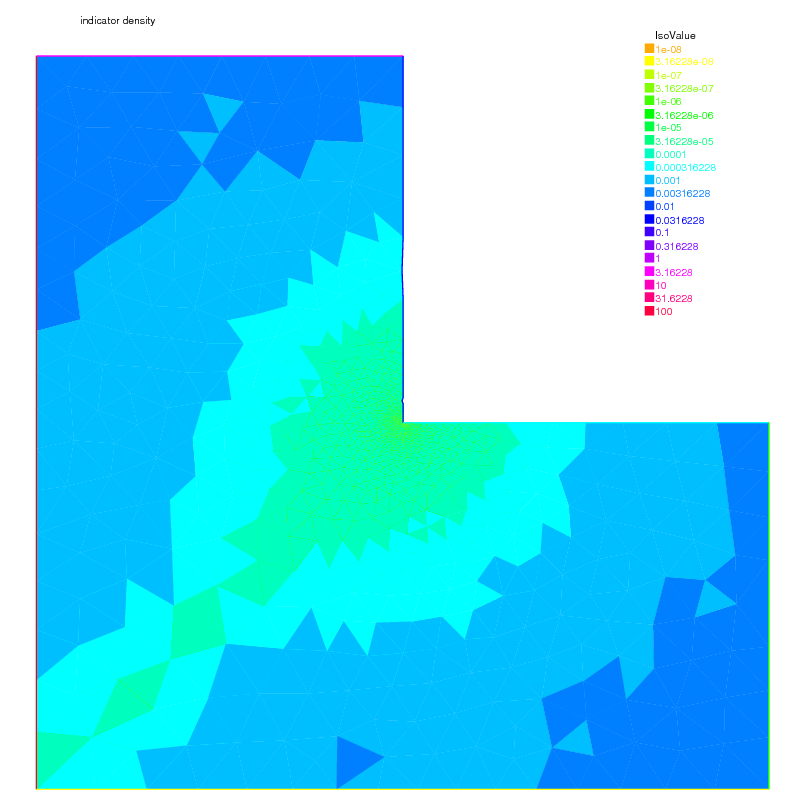Fig. 146 Density of the error indicator with isotropic $$P_{2}$$ metric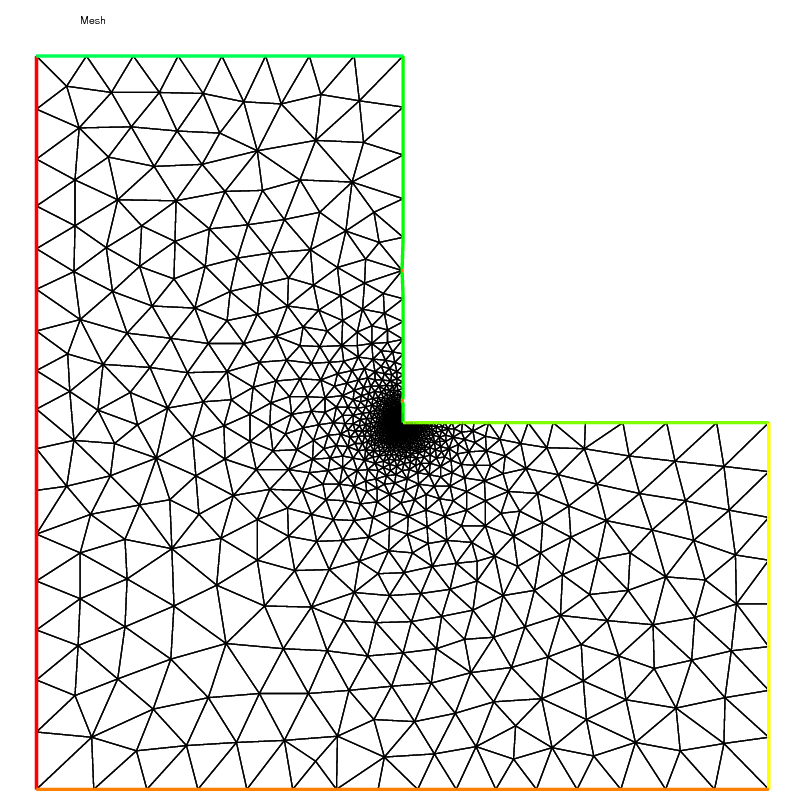Fig. 147 Density of the error indicator with isotropic $$P_{2}$$ metric

## Adaptation using residual error indicator

In the previous example we compute the error indicator, now we use it, to adapt the mesh. The new mesh size is given by the following formulae:

$h_{n+1}(x) = \frac{h_{n}(x)}{f_{n}(\eta_K(x))}$

where $$\eta_n(x)$$ is the level of error at point $$x$$ given by the local error indicator, $$h_n$$ is the previous “mesh size” field, and $$f_n$$ is a user function define by $$f_n = min(3,max(1/3,\eta_n / \eta_n^* ))$$ where $$\eta_n^* = mean(\eta_n) c$$, and $$c$$ is an user coefficient generally close to one.

First a macro MeshSizecomputation is defined to get a $$P_1$$ mesh size as the average of edge length.

 1// macro the get the current mesh size parameter
2// in:
3// Th the mesh
4// Vh P1 fespace on Th
5// out :
6// h: the Vh finite element finite set to the current mesh size
7macro MeshSizecomputation (Th, Vh, h)
8{
9    real[int] count(Th.nv);
10    /*mesh size (lenEdge = integral(e) 1 ds)*/
11    varf vmeshsizen (u, v) = intalledges(Th, qfnbpE=1)(v);
12    /*number of edges per vertex*/
13    varf vedgecount (u, v) = intalledges(Th, qfnbpE=1)(v/lenEdge);
14    /*mesh size*/
15    count = vedgecount(0, Vh);
16    h[] = 0.;
17    h[] = vmeshsizen(0, Vh);
18    cout << "count min = " << count.min << " max = " << count.max << endl;
19    h[] = h[]./count;
20    cout << "-- bound meshsize = " << h[].min << " " << h[].max << endl;
21} //


A second macro to re-mesh according to the new mesh size.

 1// macro to remesh according the de residual indicator
2// in:
3// Th the mesh
4// Ph P0 fespace on Th
5// Vh P1 fespace on Th
6// vindicator the varf to evaluate the indicator
7// coef on etameam
8macro ReMeshIndicator (Th, Ph, Vh, vindicator, coef)
9{
10    Vh h=0;
11    /*evaluate the mesh size*/
12    MeshSizecomputation(Th, Vh, h);
13    Ph etak;
14    etak[] = vindicator(0, Ph);
15    etak[] = sqrt(etak[]);
16    real etastar= coef*(etak[].sum/etak[].n);
17    cout << "etastar = " << etastar << " sum = " << etak[].sum << " " << endl;
18
19    /*etaK is discontinous*/
20    /*we use P1 L2 projection with mass lumping*/
21    Vh fn, sigma;
22    varf veta(unused, v) = int2d(Th)(etak*v);
23    varf vun(unused, v) = int2d(Th)(1*v);
24    fn[] = veta(0, Vh);
25    sigma[] = vun(0, Vh);
26    fn[] = fn[]./ sigma[];
27    fn = max(min(fn/etastar,3.),0.3333);
28
29    /*new mesh size*/
30    h = h / fn;
31    /*build the mesh*/
32    Th = adaptmesh(Th, IsMetric=1, h, splitpbedge=1, nbvx=10000);
33} //

 1// Parameters
2real hinit = 0.2; //initial mesh size
3func f=(x-y);
4
5// Mesh
6border ba(t=0, 1.0){x=t; y=0; label=1;}
7border bb(t=0, 0.5){x=1; y=t; label=2;}
8border bc(t=0, 0.5){x=1-t; y=0.5; label=3;}
9border bd(t=0.5, 1){x=0.5; y=t; label=4;}
10border be(t=0.5, 1){x=1-t; y=1; label=5;}
11border bf(t=0.0, 1){x=0; y=1-t; label=6;}
12mesh Th = buildmesh(ba(6) + bb(4) + bc(4) + bd(4) + be(4) + bf(6));
13
14// Fespace
15fespace Vh(Th, P1); //for the mesh size and solution
16Vh h = hinit; //the FE function for the mesh size
17Vh u, v;
18
19fespace Ph(Th, P0); //for the error indicator
20
21//Build a mesh with the given mesh size hinit
22Th = adaptmesh(Th, h, IsMetric=1, splitpbedge=1, nbvx=10000);
23plot(Th, wait=1);
24
25// Problem
26problem Poisson (u, v)
27    = int2d(Th, qforder=5)(
28        u*v*1.0e-10
29        + dx(u)*dx(v)
30        + dy(u)*dy(v)
31    )
32    - int2d(Th, qforder=5)(
33        f*v
34    )
35    ;
36
37varf indicator2 (unused, chiK)
38    = intalledges(Th)(
39        chiK*lenEdge*square(jump(N.x*dx(u) + N.y*dy(u)))
40    )
41    + int2d(Th)(
42        chiK*square(hTriangle*(f + dxx(u) + dyy(u)))
43    )
44    ;
45
47for (int i = 0; i < 10; i++){
48    u = u;
49
50    // Solve
51    Poisson;
52    plot(Th, u, wait=true);
53
54    real cc = 0.8;
55    if (i > 5) cc=1;
56    ReMeshIndicator(Th, Ph, Vh, indicator2, cc);
57    plot(Th, wait=true);
58}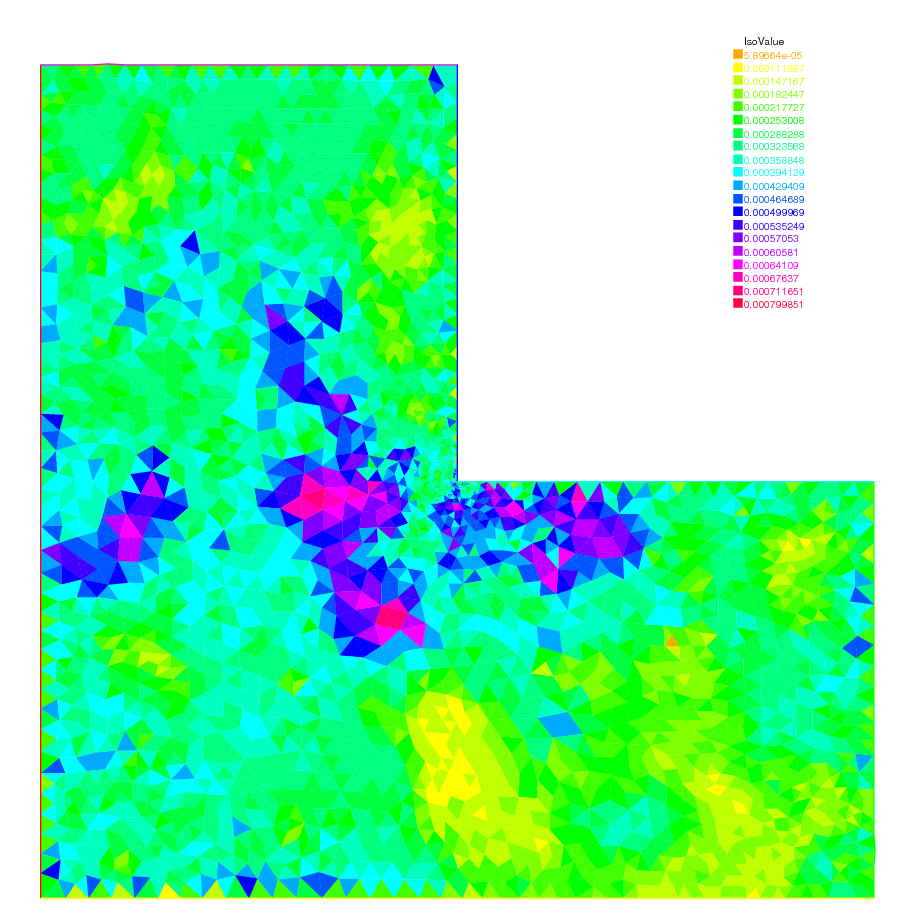Fig. 148 The error indicator with isotropic $$P_{1}$$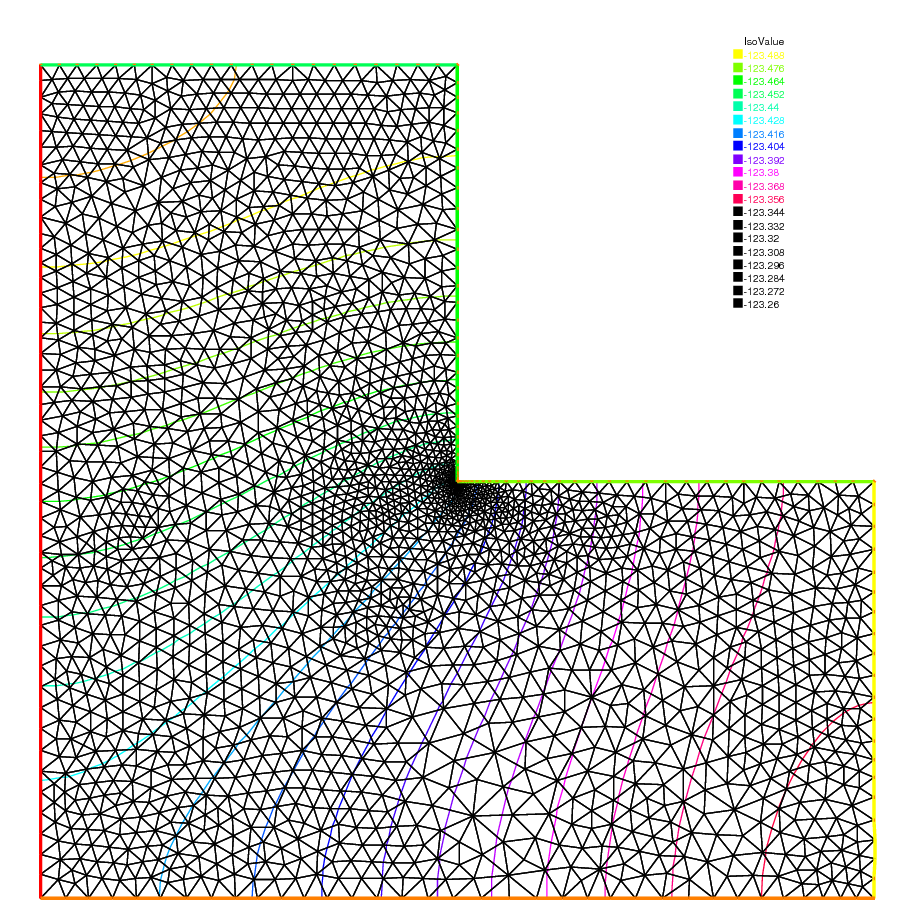Fig. 149 The mesh and isovalue of the solution